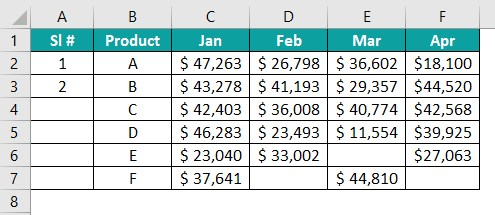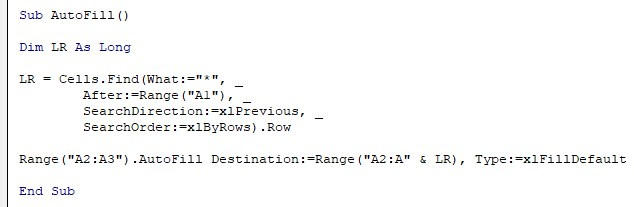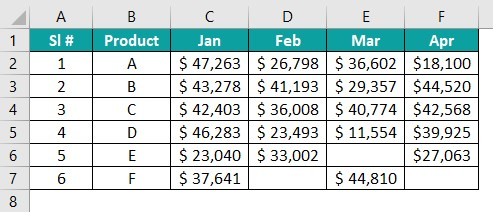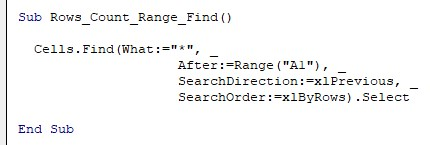# VBA Last RowArticle byJeevan A Y## Excel VBA Last Row

VBA Last row helps us find the last row in Excel. When we need to perform the same task in Excel, it is common practice to perform it within a loop (For Next, For Each). These loops need an initial and an end number to decide how many times they have to run. For this, we must find the last used cell or row of the worksheet dynamically.

In this article, we will learn all the advanced calculations and coding used to find the last used row.

###### Key Takeaways
• VBA Last row is used to find the last row in the worksheet. Finding the last row means the first non-blank cell from the bottom of the worksheet.
• To find the last row in VBA, we should use the Rows.Count method. This method will search for the first non-blank cell from the bottom of the worksheet.
• We can also select a required column and find VBA last row of that particular column using the Rows.Count method.

### How To Find Last Used Row In The Column?

We can find the last used row of the column in many ways, and we will show you some of those ways in detail now.

### 1 – Using Special Cells To Find Last Row

For your better understanding of finding the last used row in the Excel worksheet, first, we will show you the same method in the worksheet. Then, we will move on to write the VBA code.

For example, look at the following data in Excel.

Step 1: In the above data table, the last used cell is E7, to go to this cell, we need to use the shortcut keys Ctrl + End.

Select cell A1.

Step 2: Press the shortcut keys Ctrl + End to go to the last used cell of the worksheet.

This takes us to the last used cell i.e., cell E7.

Step 3: Similarly, in VBA, we can use the special cells method to find the last used cell of the given worksheet.

The following code will return the last used cell address of the worksheet.

Sub Last_Used_Row()

Dim LC As String
‘LC = Last Cell
MsgBox LC

End Sub

The above code will return the last used cell address in the message box.

As we can see, the last used cell of the worksheet is cell E7.

Step 4: In the special cell type, we have used the ‘Address’ property. To get the last used cell row number, we need to use the ROW property.

Sub Last_Used_Row()
Dim LC As String
‘LC = Last Cell
LC = Range(“A1”).SpecialCells(xlCellTypeLastCell).Row
MsgBox LC
End Sub

This will return the last used cell row number.

However, there are drawbacks to this method. Let us show you some of them with practical examples.

Step 5: The last used cell means not exactly the last non-blank cell. For example, let us delete the value in cell E7.

We just deleted the value in cell E7 and did nothing apart from this.

Step 6: Now, let’s run the code and see what would be the last used row number.

The last used row number is still row number 7.

To get the last used non-blank cell value, we need to delete cell E7 (all the formatting to be deleted) and save the workbook to get the last used row number correctly.

Now, we have deleted the value in cell E7 and saved the workbook. Next, when we run the code, we will get the last used row as 5.

### 2 – Using Rows.Count For Non-Empty Cells

To get to the last non-blank cell of any particular column, we can use the Rows.Count method. We will use the same data from the previous example.

We will find the last used cell row number using the Rows.Count option. Before we write a code to find the last used row, let us show you the activity that we do in the worksheet.

Step 1: Firstly, we need to go to the last cell of the worksheet. To go to the last cell of the worksheet, we will press the excel shortcut keys Ctrl + Down Arrow while the active cell is cell A1.

Step 2: When we use the Ctrl + Down Arrow, it takes us to the last non-blank cell i.e., A5. From this cell, again press the shortcut keys Ctrl + Down Arrow, and it will take us to the last cell of column A.

Step 3: Now, we are in the last cell of column A. From this cell, to go to the last used cell, press the shortcut keys Ctrl + Up Arrow.

It takes us to the last used cell of column A.

We will be doing a similar approach with VBA as well.

Step 4: First, define a VBA variable to hold the row number, and assign the numerical data type as Long.

Step 5: For this variable, we will assign the last row.

Step 6: Enter the Cells property and open the parenthesis.

Step 7: Now, we need to go to the last cell. To go to the last cell, use the Rows.Count option.

Rows.Count will count how many rows are there. Next, we need to provide the column number of which column we are trying to find last row in VBA i.e., column 1.

Step 8: Right now, we are in the last cell of column A. From this cell, we need to press the shortcut keys Ctrl + Up Arrow. In VBA, we can use the END property and then choose the xlUp key to move upwards.

Step 9: This will take us to the last used cell of column A. To get the row number of the last used cell, we need to use the ROW property.

Step 10: Now, show the variable LR value in the message box.

Sub Rows_Count_Last_Row ()

Dim LR As String

‘LR = Last Row

LR = Cells(Rows.Count, 1).End(xlUp).Row

MsgBox LR

End Sub

This will return the last used row number.

Step 11: To check how it works, let us add some value by leaving two empty rows.

This code will return the last used row as 9.

We can also use the Range object instead of the CELLS property to find the last used cell row. The following code is for your reference.

Sub Rows_Count_Last_Row()
Dim LR As String
‘LR = Last Row
LR = Range(“A” & Rows.Count).End(xlUp).Row
MsgBox LR
End Sub

With the RANGE object, we are providing column A as the column number, and in this column only, it will find the last used cell row.

### 3 – Using Rows.Count For Any Selected Column

The previous example returned the last used cell row number of column A. However, it is not necessary that we always need the last used cell row number of just column A. So, we must have the flexibility of finding the last used cell row number of any selected column.

The following code will return the last used cell row number of the selected column.

Sub Rows_Count_Selected_Column()

``````Dim LR As Long
'LR = Last Row
Dim C As Long
' C = Column

C = Selection.Column

LR = Cells(Rows.Count, C).End(xlUp).Row

MsgBox LR``````

End Sub

Step 1: Before we run the code, we need to select the column for which we need to find the last used row. Let us select column C.

Step 2: Now run the code.

In the selected column C, the last used row number is 6.

Likewise, we can select any of the cells and test the code.

### 4 – Using UsedRange To Find Last Row

We can also find the last used row of the active sheet. The following code will return the last used row by using the used range property.

Sub Rows_Count_UsedRange()

``````Dim LR As Long
'LR = Last Row

Dim Ws As Worksheet

Set Ws = ActiveSheet

LR = Ws.UsedRange.Rows(Ws.UsedRange.Rows.Count).Row

MsgBox LR``````

End Sub

This will return the last used cell row of the used range of the current worksheet.

### 5 – Using Range.Find To Find Last Row

The Find method in VBA is used for various tasks, and we can use it to find the last used cell row as well. The syntax of the Range.Find method is as follows:

Let us simplify the syntax for better understanding.

Now, look at the following code.

Sub Rows_Count_Range_Find()

``````Dim LR As Long
'LR = Last Row

LR = Cells.Find(What:="*", _
After:=Range("A1"), _
SearchDirection:=xlPrevious, _
SearchOrder:=xlByRows).Row
MsgBox LR``````

End Sub

Code Explanation:

The above looks for the first non-blank cell in the entire worksheet starting from cell A1 but searches from the bottom of the worksheet.

What:= “*” – In the What syntax of the Find method, we are using the wildcard character asterisk (*) that finds any text or number in the cell. In simple terms, it looks for the first non-blank cell.

After:= Range(“A1”) – The search should start from cell A1 which is the first cell in the worksheet. Since we are providing the search to happen after cell A1, the find will not be taking cell A1 into consideration. Find will operate only after cell A1 depending upon the SearchOrder and SearchDirection.

SearchDirection: = xlPrevious – This argument is important because, in this argument, we provided the input as xlPrevious i.e., the find should start from right to left and bottom to top.

SearchOrder: = xlByRows – This allows the find to happen on a row-by-row basis.

Let us show you some examples now to understand the functionality.

Look at the following data.

When we run this code, we will get the last used row as 7.

Now add some value in cell A10.

Now run the code, and it should return row number 10 as the last used row.

Now add some value in cell D12.

Now run the code and see what happens.

Now it is returning the last used cell row as 12.

This is the best part of Range.Find method. It finds the last used cell which has a value.

Range.The find method is more versatile than the previous methods.

In the Rows.Count method, we need to specify the column number for which we are trying to find the row number. But with the Find method, we need not have to provide the column number.

### Important Things To Note

• With the special cell method, Excel only resets the last cell when the workbook is saved.
• The special cells method finds the last used cell, not the last non-blank cell.
• Ctrl + End are the shortcut keys in the worksheet to go to the last used cell.
• Address property will return the cell address, whereas Row property will return the row number of the last used cell.
• Find method search direction should be xlPrevious otherwise, it will find the first non-blank cell from the top of the worksheet.

1. How to autofill to the VBA last row?

To auto-fill the value, we need to find the last used to limit the auto-fill functionality. For example, look at the following data.In column A, we need to insert serial numbers starting from cell A2, and the serial number should be incremented by 1.

We have entered the values 1 and 2 in cells A2 and A3, respectively. The following code will auto-fill numbers incremented by 1 till the last used row.This will auto-fill numbers till the last used cell row.2. How to go and select VBA last row?

For example, look at the following data in Excel.To select the last used cell, we can use the following code.This will select the last used cell of the worksheet.

3. Why is VBA Last Row Not Working?

To avoid errors, always provide a row property at the end to get the row number of the last used cell.